# Text Functions

Excel includes functions for textual strings to reformat, separate, combine, and rewrite the text. These functions can be combined together to do advanced actions when working with textual strings.

In this chapter, we will reference data in Figure 32.1 that includes a City, State, Zip Code text string, and Population and Rank data. However, we only need the former text string.

Use this workbook for the chapter.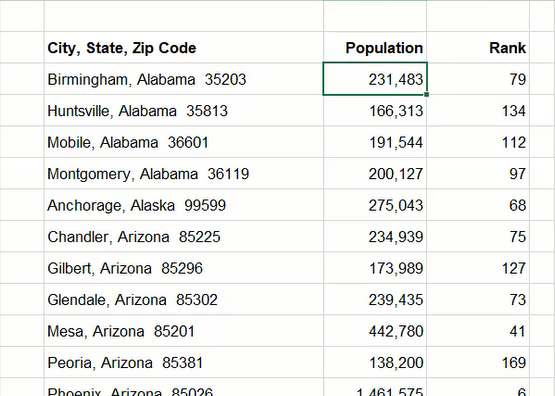Figure 32.1

For ease of reference, it is recommended to freeze our top row(s) containing headers and the left column(s) containing the City, State, Zip Code information. We can do this by selecting the cell below the header row and right of the aforementioned column, then going to the View tab of the ribbon toolbar, and selecting Freeze Panes and Freeze Panes again.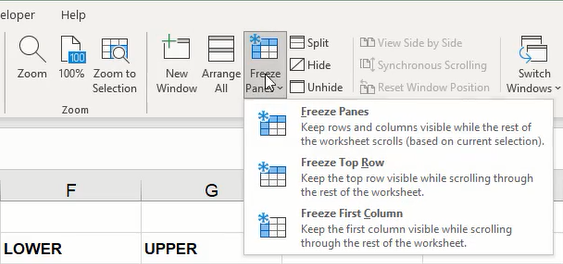Figure 32.2

## Lower

The Lower function is used to edit a text string to have all lowercase letters. In this example, we will use it to lowercase all letters in the city, state, and zip code information cell.

1. Type =LOWER and a left parenthesis ( ( ) to start the function.
2. Select the cell containing the City, State, Zip Code data.
3. Type a right parenthesis ( ) ) to close the formula.
4. Press Enter to complete the function. (See Figure 32.3)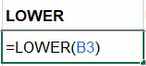Figure 32.3

## Upper

The Upper function is used to edit a text string to have all uppercase letters. We can use it to uppercase all letters in the city, state, and zip code information cell.

1. Type =UPPER and a left parenthesis ( ( ) to start the function.
2. Select the cell containing the City, State, Zip Code data.
3. Type a right parenthesis ( ) ) to close the formula.
4. Press Enter to complete the function. (See Figure 32.4)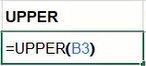Figure 32.4

## Proper

The Proper function is used to edit a text string to uppercase the first letter of each word. Our original reference data already has words formatted in this manner, but we can use one of the previously completed Lower or Upper function cells for reference.

1. Type =PROPER and a left parenthesis ( ( ) to start the function.
2. Select one of the previous function cells containing all lower or upper case text.
3. Type a right parenthesis ( ) ) to close the formula.
4. Press Enter to complete the function. (See Figure 32.5)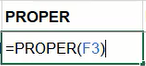Figure 32.5

## Left

The Left function can be used to pull and display a designated number of characters beginning from the left of a text string.

1. Type =LEFT and a left parenthesis ( ( ) to start the function.
2. Select the cell containing the City, State, Zip Code data and type a comma ( , ).
3. Type a preferred number of characters to pull from the selected cell.
1. In this example, we'll pull three characters from the text string of the selected cell.
4. Type a right parenthesis ( ) ) to close the formula.
5. Press Enter to complete the function. (See Figure 32.6)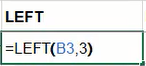Figure 32.6

## Right

Identical to the Left function, the Right function can be used to pull and display a designated number of textual characters beginning from the right of a text string. For this example, we'll pull five characters to copy the zip code at the end of the text string.

1. Type =RIGHT and a left parenthesis ( ( ) to start the function.
2. Select the cell containing the City, State, Zip Code data and type a comma ( , ).
3. Type a preferred number of characters to pull from the selected cell.
1. In this example, we'll pull five characters from the text string of the selected cell.
4. Type a right parenthesis ( ) ) to close the formula.
5. Press Enter to complete the function. (See Figure 32.7)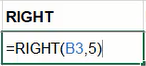Figure 32.7

## Length

The Length function counts the number of characters in a cell's text string.

1. Type =LEN and a left parenthesis ( ( ) to start the function.
2. Select the preferred cell to evaluate the number of characters it contains.
3. Type a right parenthesis ( ) ) to close the formula.
4. Press Enter to complete the function. (See Figure 32.8)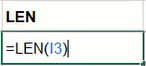Figure 32.8

## Trim

The Trim function removes any extra spaces found within the cell's text string. The data for city, state, and zip code includes two spaces between state and zip code information. We can use Trim to remove the second space.

1. Type =TRIM and a left parenthesis ( ( ) to start the function.
2. Select the cell containing the City, State, Zip Code data.
3. Type a right parenthesis ( ) ) to close the formula.
4. Press Enter to complete the function. (See Figure 32.9)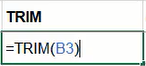Figure 32.9

## Ampersand

An ampersand symbol instructs Excel to combine two references in an argument.

1. Type an equals sign ( = ).
2. Select the first cell to be combined and type an ampersand ( & ).
3. Type " " (with a space between the quotation marks).
4. Type an ampersand ( & ) and select the second cell to be combined.
5. Press Enter to complete the formula. (See Figure 32.10)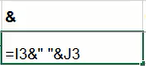Figure 32.10

## Concatenate

Similar in function to the ampersand, the CONCAT (Concatenate) function combines multiple cell references into one cell.

1. Type =CONCAT and a left parenthesis ( ( ) to start the function.
2. Select the first cell to be combined and type a comma ( , ).
3. Select a second cell to be combined.
1. Additional cells may be combined by typing another comma and selecting the next cell.
4. Type a right parenthesis ( ) ) to close the formula.
5. Press Enter to complete the function. (See Figure 32.11)

Note: Like the previous function example, you may need to include a space between quotation marks to separate the selected items. For example, the Figure 32.11 formula would look like the following: =CONCAT(M3," ",K3," ",J3)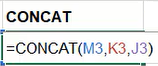Figure 32.11

## Value

The Value function converts numbers used in a textual string format to number format. In the case of the zip code produced in the earlier Right function section, we'll use Value combined with Right to convert the zip code into a numerical format whereby it will be right-aligned in the cell.

1. Type =VALUE and a left parenthesis ( ( ) to start the function.
2. Type RIGHT and a second left parenthesis ( ( ).
3. Select the previous Right function cell containing the zip code data.
4. Type two right parenthesis ( ) ) to close both formulas.
5. Press Enter to complete the function. (See Figure 32.12)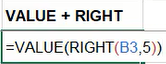Figure 32.12

## Find

The Find function is used to locate the first position of an indicated character in a referenced textual string.
Note: This function is case-sensitive.

1. Type =FIND and a left parenthesis ( ( ) to start the function.
2. Type "," and a comma ( , ).
3. Select the cell containing the City, State, Zip Code data.
4. Type a right parenthesis ( ) ) to close the formula.
5. Press Enter to complete the function. (See Figure 32.13)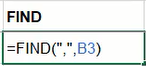Figure 32.13

## Search

Identical to the Find function, the Search function will locate the first position of an indicated character in a text string.
Note: This function is not case-sensitive.

1. Type =SEARCH and a left parenthesis ( ( ) to start the function.
2. Type "a" and a comma ( , ).
3. Select the cell containing the City, State, Zip Code data.
4. Type a right parenthesis ( ) ) to close the formula.
5. Press Enter to complete the function. (See Figure 32.14)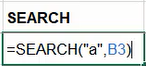Figure 32.14

## Replace

The Replace function is capable of rewriting a designated portion of the referenced cell's text. In this example, we'll reference the city, state, and zip code cell, indicate a starting point, determine the number of characters from that point to be replaced, and insert a new string.

1. Type =REPLACE and a left parenthesis ( ( ) to start the function.
2. Select the cell containing the City, State, Zip Code data and type a comma ( , ).
3. Type 1 to indicate the beginning of the text string.
4. Type a comma ( , ).
5. Type 10 or another number to indicate the number of characters to replace.
6. Type the new text enclosed in double quotation marks.
1. In this example, we will use "Example" for the new text.
7. Type a right parenthesis ( ) ) to close the formula.
8. Press Enter to complete the function. (See Figure 32.15)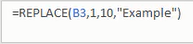Figure 32.15

## Substitute

Substitute works similarly to the Replace function by searching for a designated character or text to be substituted and inserting the new text.

1. Type =SUBSTITUTE and a left parenthesis ( ( ) to start the function.
2. Select the cell containing the City, State, Zip Code data and type a comma ( , ).
3. Type "a" and a comma ( , ).
4. Type "_" to indicate the new character.
5. Type a right parenthesis ( ) ) to close the formula.
6. Press Enter to complete the function. (See Figure 32.16)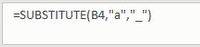Figure 32.16

## Middle

The Middle function works like the Left and Right functions by beginning at a specified position in a text string and pulling a determined number of characters.

1. Type =MID and a left parenthesis ( ( ) to start the function.
2. Select the cell containing the City, State, Zip Code data and type a comma ( , ).
3. Type the preferred number of characters to indicate the start point and a comma ( , ).
4. Type the preferred number of characters to pull from the selected cell.
5. Type a right parenthesis ( ) ) to close the formula.
6. Press Enter to complete the function. (See Figure 32.17)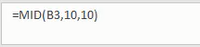Figure 32.17

Now, we want to extract individual data from the City, State, Zip Code column. We will start by finding the city using the =LEFT function, then the state using the =MID function, and finally, the zip code using the =RIGHT function.

## Combining Functions

The City column will be our first step. In this column, we’ll be using the =LEFT function. Use the following steps to extrapolate the city from the City, State, Zip Code column:,

1. Type =LEFT and a left parenthesis ( ( ) to start the function.
2. Select the cell containing the City, State, Zip Code data and type a comma ( , ).
3. Type FIND and a second left parenthesis ( ( ).
4. Type "," and a comma ( , ).
5. Select the same cell containing City, State, Zip Code data.
6. Type a right parenthesis ( ) ) to close the FIND formula.
7. Type -1 or another number to subtract extra characters as needed.
8. Type a right parenthesis ( ) ) to close the LEFT formula.
9. Press Enter to complete the function. (See Figure 32.18)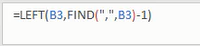Figure 32.18

The State column is a little trickier. In this column, we’ll be using the =MID function. Use the following steps to extrapolate the state from the City, State, Zip Code column:

1. Type =MID and a left parenthesis ( ( ) to start the function.
2. Select the cell containing the City, State, Zip Code data and type a comma ( , ).
3. Type LEN and a second left parenthesis ( ( ).
4. Select the previous cell containing only the city name.
5. Type a right parenthesis ( ) ) to close the LEN formula.
6. Type +3 or another number to add extra characters as needed.
7. Type a comma ( , ).
8. Type FIND and a left parenthesis ( ( ).
9. Type "  " (with two spaces between the quotation marks) and a comma ( , ).
10. Select the same cell containing City, State, Zip Code data.
11. Type a right parenthesis ( ) ) to close the FIND formula.
12. Type -LEN and a left parenthesis ( ( ).
13. Select the previous cell containing only the city name.
14. Type a right parenthesis ( ) ) to close the LEN function.
15. Type -3 or another number to subtract extra characters as needed.
16. Type a right parenthesis ( ) ) to close the MID formula.
17. Press Enter to complete the function. (See Figure 32.19)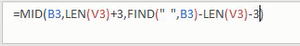Figure 32.19

If you ever want to make sure you have those extra spaces removed, you can always wrap the entire formula in a trim function, which will remove the extra spaces for you. (See Figure 32.20)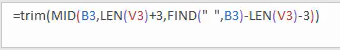Figure 32.20

The Zip Code will be the most straightforward step. In this column, we’ll be using the =RIGHT function. Use the following steps to extrapolate the zip code from the City, State, Zip Code column:

1. Type =RIGHT and a left parenthesis ( ( ) to start the function.
2. Select the cell containing the City, State, Zip Code data and type a comma ( , ).
3. We only want the five characters from the right of the cell, so type the number 5.
4. Embed the =RIGHT formula in a value by typing value( between the equal sign ( = ) and RIGHT.
5. Add a left parenthesis ( ( ) after value to embed the function.
6. Close the formula with a right parenthesis ( ) ) at the end. See Figure 32.21 for a completed formula.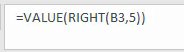Figure 32.21

Now that you have the City, State, and Zip Code in separate columns, select all three input cells and click and drag down to automatically update the columns. It will even account for spaces between words, like Little Rock

These text functions are invaluable when manipulating data; take the time to learn them to manipulate text quickly and accurately.